# Generation of Electrical Oscillations

The following article is from The Great Soviet Encyclopedia (1979). It might be outdated or ideologically biased.

## Generation of Electrical Oscillations

the process of converting various forms of electrical energy into the energy of electrical (electromagnetic) oscillations. The term is usually applied to oscillations in the radio-frequency range, which are excited in equipment (systems) with lumped parameters (capacitance C, inductance L, and resistance R) where the electrical and magnetic fields are spatially separated. At high frequencies (superhigh frequency and the optical band), systems with distributed parameters are necessary to excite oscillations. In this case one speaks of electromagnetic oscillations. The term “generation of electrical oscillations” is not generally used when referring to the production of alternating current at industrial frequencies by means of electrical machines.

Electrical oscillations are usually generated either by converting energy from DC sources by means of electronic devices (vacuum, gas-discharge, or solid-state) or by converting primary electrical oscillations into oscillations with the required frequency and shape (parametric generators and quantum generators).

The process of generation is distinguished according to the type of electronic devices used: vacuum-tube oscillators (using electron vacuum tubes), semiconductor oscillators (with crystal triodes, tunnel diodes, and so on), and oscillators with gas-discharge devices (thyratrons and others). It is also classified according to the shape of the oscillations, the frequency, the power, and the purpose: oscillators of sinusoidal (harmonic) oscillations, oscillators of specially shaped oscillations, superhigh-frequency oscillators, and so on.

The necessary elements of an oscillator are an energy source, a circuit in which the oscillations are produced and maintained (passive circuits), and an active element, which converts the energy from the source into oscillatory energy. The active element is usually an electronic device, frequently in combination with auxiliary control (feedback) circuits.

If the energy supplied to the passive circuits exceeds the energy losses in the circuits, then any oscillatory process that occurs in them will increase. If the inflow is less than the losses, the oscillations will die out. An energy equilibrium corresponding to a steady-state generation of electrical oscillations can only be achieved when the system’s elements have nonlinear properties. Without such properties the oscillatory process in the system either increases or decays, and the generation of steady-state electrical oscillations is impossible.

If the circuits in which oscillatory processes are produced and maintained have themselves oscillatory characteristics (for example, an oscillatory circuit or a cavity resonator), the frequency and shape of the oscillations generated is mainly determined by the frequency and shape of their natural oscillations. In this case the part played by the active element is reduced to merely pumping energy into the circuit to compensate for losses (including the energy taken off by the user).

Generators of quasi-harmonic oscillations. If the circuit or resonator losses are small in a generator with oscillatory circuits (high quality of the oscillatory system), the shape of their oscillations will be nearly sinusoidal and they are known as quasi-harmonic or Thomson oscillation generators.

TUBE OSCILLATOR. The simplest vacuum-tube generator of quasi-harmonic oscillations consists of an oscillatory circuit and an electron vacuum tube (for example, a triode) with a supply and a control circuit (Figure 1). In response to random electrical oscillations in the circuit, natural current and voltage oscillations develop. However, because of energy losses in the oscillatory circuit, the oscillations would die out. To prevent this it is necessary to replenish the supply of oscillatory energy in the circuit—for example, by reaching it with a pulsating current of the same frequency and appropriate phase; this is achieved with a triode. The varying voltage supplied to its grid from the circuit produces a change in its plate current. As a result, pulsations occur in the plate current which, with a proper choice of the phase for the voltage supplied to the tube’s grid (the feedback circuit), will replenish the circuit’s oscillatory energy.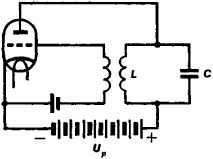Figure 1. simplest vaccum-tube oscillator for quasi-harmonic oscillations: LC is the oscillatory circuit (C is a capacitance, and L is an inductance), and Up is the plate

If the tube’s amplification is such that the replenished oscillatory energy exceeds the losses of the oscillatory circuit during the same period, the amplitude of the original oscillations produced in the circuit will increase. As the oscillation amplitude increases, the tube’s amplification decreases because of nonlinearity in the triode’s current-voltage characteristic, and a steady amplitude of the oscillations generated in the system is established. Such systems, which generate steady-state oscillations whose frequency and shape are determined by the properties of the system itself, are called self-oscillating systems or self-excited oscillators, and the oscillations they generate are self-excited oscillations.

The power supplied from the source is expended not only in maintaining oscillations in the circuit but also in heating the plate of the tube by the electrons that bombard it when plate current is flowing. This limits the efficiency of tube generators, which can nevertheless reach 70-75 percent.

The electron vacuum tube may be controlled in various ways by means of a feedback circuit. In addition to inductive feedback (Figure 1), capacitive feedback (Figure 2,a) and autoinductive feedback (Figure 2,b) are also possible.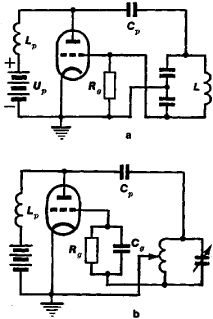Figure 2. Generators with (a) capacitive and (b) autoinductive feedback

In vacuum-tube generator circuits the so-called parallel plate supply circuit (Figure 2, a and b) and automatic bias, created by the grid current ig, are often used. The current ig develops a constant voltage on the tube’s control grid that shifts the operating point on the anode-grid characteristic into the region of negative values needed for a high efficiency (Figure 3).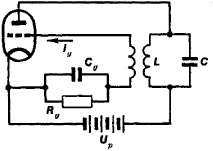Figure 3. Diagram of a vaccum tube oscillator with automatic grid bias

The power of vacuum-tube generators runs from fractions of a watt (in measuring and calibrating apparatus) up to dozens and hundreds of kilowatts; the frequencies generated range from dozens of kilohertz (kHz) to a gigahertz (GHz). The upper frequency limit is due first to the presence of spurious capacitances (grid-plate and others) in the vacuum tubes and to the finite transit time of electrons from cathode to plate, as well as to certain other factors. The lower frequency limit results from the low quality of oscillatory circuits at low natural frequencies.

TRANSISTOR GENERATOR. Another example of a quasi-harmonic oscillation generator is an oscillator using a semiconductor triode (a transistor generator). Here, as in the vacuum-tube oscillator, there is a power source, a high-quality oscillatory circuit, and an active element consisting of a semiconductor triode combined with a feedback circuit. In semiconductor triodes (transistors) the oscillations supplied to a control electrode (for example, to the base) are amplified in power, and this, as with electron vacuum tubes, makes it possible to pump oscillatory energy into the circuit by means of a feedback circuit in order to produce and maintain a condition of steady-state (sustained) oscillations. There are various transistor generator circuits. Three versions of semiconductor generators that use the common-emitter transistor circuit connection are shown in Figure 4, a, b, and c.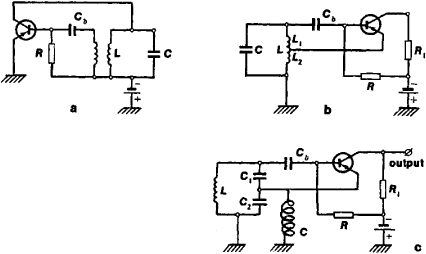Figure 4. Transistor generators using junction triodes with (a) inductive, and (c) capactive feedback; c is a choke

Transistor generators produce oscillations having frequencies ranging from several kHz up to 1010 Hz, with power from tenths of a milliwatt to hundreds of watts. Like vacuum-tube generators, when the quality of the circuit is high, the shape of the oscillations is nearly harmonic and the frequency is controlled by the natural frequency of the oscillatory circuit, taking into account the transistor’s spurious capacitances.

NEGATIVE DIFFERENTIAL RESISTANCE. The production of sustained oscillations in a circuit can be regarded as the result of the insertion into it of some “negative” resistance, which compensates for the positive resistance. In a vacuum-tube oscillator, this negative resistance is created by the tube in combination with the feedback circuit and the power source. In a negative resistance a current increase must result in a decrease of the voltage drop: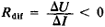(in ordinary resistances R = ΔUI > 0). A negative differential resistor effect only occurs when the amplifying properties of a tube or transistor are used because of positive feedback.

However, there are devices in which the current-voltage characteristics have a decreasing section under certain conditions. This means that in a certain range of values of U and I, differential resistance is negative, Rdif = ΔUI < 0 (Figure 5), thus permitting such devices to be used to generate oscillations. For example, in pentodes the relationship between the screen grid current is and the suppressor grid voltage Usup has a decreasing section (Figure 6,a). This negative resistance makes it possible to construct the so-called transitron generator (Figure 6,b). The oscillations in the LC circuit of this generator are also maintained by the negative resistance introduced into the circuit through the action of the tube’s screen grid current, which is controlled by the voltage on the third suppressor grid.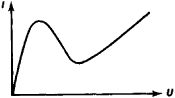Figure 5. Current-voltage characteristic with a decreasing section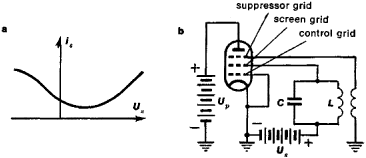Figure 6. (a) Screen grid current in a pentode as a function of its suppressor grid voltage, (b) transition generator circuit

A negative resistance can also be created by utilizing an electrical discharge in gases that have a decreasing section in their current-voltage characteristic. For example, in certain modes of arc discharge, the arc temperature rises with an increase in the current i, thus increasing the number of ions in the discharge gap, with a resultant decrease of the resistance and the voltage drop U between the electrodes. This property of an arch discharge was utilized in high-frequency arc generators that were in use before the appearance of vacuum-tube oscillators (Figure 7, a and b).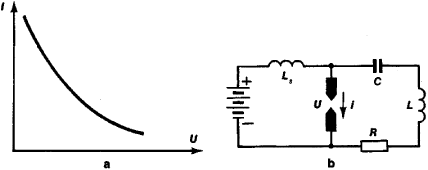Figure 7. (a) Current-voltage characteristic of an electric arc, (b) arc generator circuit

It is likewise possible to utilize the decreasing characteristic of a tunnel diode (TD; Figure 8,a). If the operating point on the diode’s characteristic is located in the decreasing section, this then corresponds to the introduction of a negative resistance into the oscillatory circuit.

With a high-quality oscillatory circuit the oscillations generated are nearly harmonic in shape, and their frequency is controlled by the natural frequency of the circuit, with allowances for the extra capacity of the diode (connected in parallel with the basic capacitance C, Figure 8). The amplitude of the steady-state oscillations will be determined by the condition that the average slope of the operating section of the characteristic (taking into account the overshoot of the oscillations beyond the limits of the steepest part of the decreasing characteristic) must provide full compensation for the losses in the circuit’s resistance, including the total generator load Rtot. In this case Rneg = Rtot.

Tunnel diode generators can produce oscillations up to frequencies of 100 GHz, but with very low power (on the order of fractions of a microwatt). For decimeter and centimeter wavelengths the power of such generators can be several milliwatts. Since they are extremely compact and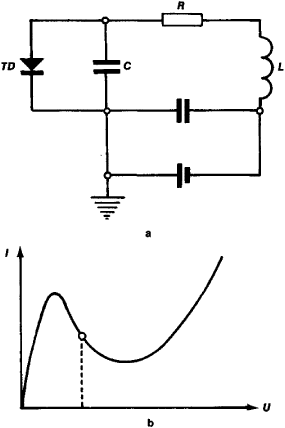Figure 8. (a) Tunnel-diode (TD) generators, (b) current-voltage characteristic of a tunnel-diode

economical, they are used most successfully as heterodyne oscillators in superhigh-frequency radio receivers. Semiconductor generators, like vacuum-tube generators, cannot generate very high frequencies (in the centimeter and shorter wavelength regions). In this frequency range, devices with cavity resonators instead of circuits are generally used.

The majority of the concepts presented above (active element, passive circuits, negative resistance, and so on) apply fully only to apparatus consisting of lumped elements (tube, resistor, capacitor, inductor, and so on) whose dimensions are considerably smaller than the wavelength λ. The advance into the superhigh-frequency region led to the development of generators that are systems with distributed parameters. In these devices, various phenomena that occur in electron beams in a vacuum, in plasma, or when a current is passed through certain solids such as semiconductors are used to generate electrical oscillations. The concept of an electrical circuit is not always applicable to these cases and it is impossible to distinguish the passive circuits and the active element.

MAGNETRON. In a magnetron, superhigh-frequency oscillations are produced in a system of cavity resonators (cavities with conducting walls). The resonators are positioned around a massive anode, and their natural frequency v is controlled by the diameter of the cavity and the width of a slot that connects each cavity with a common space that has a cathode at its center (Figure 9). A magnetic field, which bends the trajectories of electrons moving from the cathode C to the anode A, forms a common electron beam that drifts successively around the resonator slots. The magnetic field is selected so that the majority of electrons move along trajectories that almost touch the slots. Since weak electrical oscillations are unavoidable in the resonators because of random currents, weak alternating electric fields E exist around the slots. The electrons drifting in these fields are either accelerated, thus removing energy from a resonator, or are retarded, thus giving up part of the energy to the resonators, depending on their direction. Electrons that are accelerated by the field of the first resonator are returned to the cathode. The retarded (working) electrons enter the field of the next resonators, where they are also retarded if they enter during the “retarding” half-period of the electromagnetic field. By suitable selection of the electron velocities (anode voltage Ua and magnetic field H), the electrons can be made to give up more energy to the resonators than they remove from them. The oscillations in the resonators will then increase. The non-linearity of the magnetron characteristic ensures that the oscillations generated will have a constant amplitude. Energy can be removed from any resonator by means of a coupling loop L.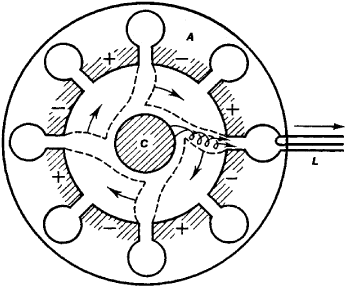Figure 9. Magnetron: A is the anode, C is the cathode, and L is the coupling loop

In a magnetron, the power supply is the source of the anode voltage Ua, and the oscillatory system is the resonator. The role of the active element that converts direct current energy into the energy of electrical oscillations is played by the electron stream, which is subject to the magnetic field.

Magnetrons generate harmonic oscillations in the frequency range from 300 MHz to 300 GHz, with an efficiency of up to 85 percent. They are usually used to produce high-power oscillations (several megawatts) in the pulsed mode and several dozen kilowatts in the continuous mode.

KLYSTRON. A klystron also contains a cavity resonator in which oscillations are produced and maintained by an electron stream. The stream from cathode C (Figure 10,a) is accelerated by an electric field created by the power source. In a reflex klystron the electrons pass through the grids G of a cavity resonator (without reaching the anode A, whose potential is negative with respect to the resonator grids), are reflected, pass through the resonator in the opposite direction, and so on. If the electrons passed through the resonator in an unbroken stream, they would then give up energy to the resonators during one half-period of the resonator’s oscillations and would remove the same amount of energy during the second half-period, so that it would be impossible to generate electrical oscillations. However, if the electrons pass into the resonator in separate “bunches” at those moments when the resonator is retarding them, they give up more energy to the resonator than they remove. Here the electron stream amplifies the random oscillations that occur in the resonator and maintains them at a constant amplitude. Because the grouping of the electron stream into bunches takes place during a time that corresponds to several periods of the oscillations, the length of the “buncher space” is determined by the velocity of the electrons and the frequency of the oscillations generated. As a result, most klystron oscillators are in the centimeter and millimeter wavelength ranges. Their power is low, ranging from several milliwatts in the millimeter range to several watts in the centimeter range. The power of double-cavity drift klystrons (Figure 10,b) can reach dozens of watts in the centimeter range.

QUANTUM BEAM GENERATORS. The role of the high-quality oscillatory system in quantum generators is played by excited atoms or molecules of an active substance. In shifting from the excited state to the unexcited state, they radiate batches (quanta) of electromagnetic energy equal to hv, where h is Planck’s constant and v is the frequency of the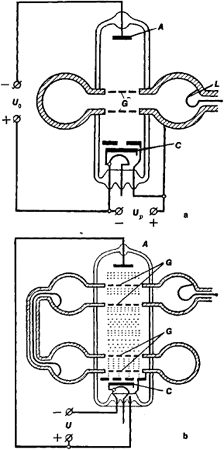Figure 10. klystrons: (a) reflex klystron, (b) double-cavity drift klystron: (G) resonator grid, (A) anode, (C) cathode

electromagnetic oscillations that are characteristic for the given type of atoms. The source of energy is the excited atoms and molecules, and a state selection system serves to choose the excited molecules. For example, in a molecular oscillator using ammonia, the power source is the source of a molecular ammonia beam. A cavity resonator in which the active substance is placed performs the feedback function, causing by means of an electromagnetic field induced radiation by the molecules and contributing oscillatory energy to compensate for the losses, including the external removal of energy. The ammonia generator operates at a frequency of 23.870 GHz with a very stable and narrow spectral line for the generated oscillations because of the high quality of the quantum transition. The high frequency stability of the oscillations produced by such generators in the radio band (operating on ammonia, hydrogen, hydrocyanic acid, and so on) permits their use as quantum frequency standards.

Relaxation generators. There is an extensive class of generators in which the passive circuits where oscillations are produced and maintained have no oscillatory properties (circuits having high losses and other aperiodic circuits—for example, combinations of capacitors C and resistors R or inductances L and resistors R). In such generators most of the oscillatory energy is lost and replaced during each period of the oscillations. The period in this case is determined by the relaxation time (the time of the process of establishing equilibrium) in these circuits—hence the name relaxation generators. The shape of the oscillations is controlled jointly by the properties of the oscillatory circuits and the active element and may vary widely—from jumplike, almost continuous oscillations (for example, multivibrators) to oscillations that are as close as desired to harmonic (RC generators for sinusoidal oscillations). This characteristic of relaxation generators is generally utilized to produce electrical oscillations of special shapes—for example, rectilinear pulses and sawtooth voltages and currents (Figure 11)—as well as to generate audio and ultralow-frequency harmonic oscillations.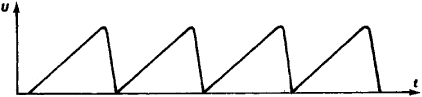Figure 11. Sawtooth voltage

THYRATRON. The simplest relaxation generator is the thyratron for sawtooth voltage (Figure 12,a). In a thyratron the ignition voltage is higher than the extinction voltage. Its voltage U varies virtually linearly with time up to some maximum value and then falls rather rapidly to the initial value (Figure 11). Since a thyratron’s current-voltage characteristic has a decreasing section (Figure 12,b), the process of charging the capacitor C up to the ignition voltage of the thyratron takes place slowly, and the accumulated charge is then quickly discharged through the thyratron; the voltage across the capacitor drops to the value at which the thyratron is extinguished. The internal resistance of the thyratron then becomes high, so that the capacitor is again charged, and so on. The oscillation period is controlled by the charge and discharge times of the capacitor—that is, by the relaxation time of an RC circuit.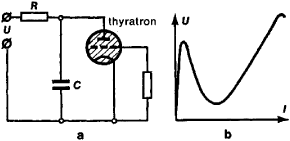Figure 12. (a)Thyratron, (b) current-voltage characteristic of a thyratron

The voltage variation across the capacitance can be made highly linear by using in place of a resistor R in the generator circuit a device (such as a pentode) that maintains a constant current during the capacitor-charging process, or by utilizing negative feedback. The oscillation frequency of a thyratron generator can be controlled within certain limits by supplying a synchronizing voltage to the thyratron’s grid.

During one period of the oscillations in this type of generator there is a complete energy exchange. All the energy stored in the capacitor during the charging time is expended through the thyratron during the discharge period. There are no circuits in which oscillatory processes can occur without power sources.

MULTIVIBRATOR. A multivibrator using electron tubes or transistors is a two-cycle device in which electrical oscillations are generated by alternately charging and discharging two capacitors C1 and C2 in the RC circuits by means of two coupled tubes (or transistors). In a symmetrical multivibrator (Figure 13,a), transistors T1 and T2 are alternately opened and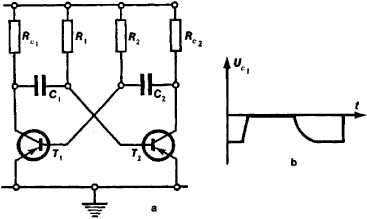Figure 13. Multivibrat using transistors T1 and T2: (a) circuit, (b) shape of oscillations

closed so that capacitors C1 and C2 are alternately charged and discharged. The abrupt changes in voltages and currents in the separate circuit elements correspond to opening and closing of the transistors (Figure 13,b). However, these rapid processes proceed in such a way that the energy stored in a capacitor changes continuously.

Various versions of multivibrators are used to produce periodic voltages of the various shapes that are required for electrical apparatus. The period of the oscillations is controlled by the relaxation times of the circuits that include the transistors. Oscillations are possible only by maintaining in the system the continually changing processes of charging and discharging the RC circuits, which do not have natural oscillatory characteristics.

RC GENERATOR. An RC generator for sinusoidal oscillations also does not include oscillatory circuits, but because of the choice of a control circuit for the active element (electron tube or transistor), the conditions necessary for generating electrical oscillations are met for only one harmonic oscillation at a frequency determined by the relaxation time of the RC network (Figure 14). For example, in an RC generator with an electron tube, a thermistor keeps the tube’s gain at a level just slightly above the critical level corresponding to self-excitation. As the current increases, the thermistor’s temperature rises and increases its resistance which, in turn, reduces the tube’s transconductance through negative feedback. Since in this case the tube is operating virtually on the linear section of its characteristic, the conditions for generating electrical oscillations will be met only at one frequency.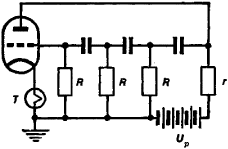Figure 14.RC generator for sinusoidal oscillations: T is a thermistor, and r is a load resistor

In such a system a complete energy exchange occurs during every oscillation period. When the power source is disconnected, the oscillations disappear and only aperiodic relaxation processes take place in the system. Harmonic oscillations are produced in RC generators over a frequency range from fractions of a hertz up to dozens and hundreds of kilohertz. RC generators are widely used as standard oscillation sources.

GUNN GENERATOR. A Gunn generator is a small (about 100 microns) semiconducting single crystal through which a direct current is passed. With current densities that produce a field intensity of not less than 3 kilovolts per cm in the semiconductor, transient processes occur within the semiconductor’s volume that result in the production of a superhigh-frequency alternating component in the current flowing through it and a superhigh-frequency voltage at its electrodes (the Gunn effect).

In a Gunn generator the energy of a direct current source is converted into oscillatory energy within the crystal, which plays a role both as an oscillatory system and as an active element. The lack of a high-quality resonator can account for the nonmonochromatic nature of the oscillations. The spectral line corresponding to the main frequency is broad. In addition, a large number of side frequencies are produced. Using Gunn generators as low-power heterodyne oscillators, electrical oscillations can be generated at frequencies from 100 MHz up to 10 GHz with a power of up to 10 MW (with continuous generation) or hundreds of watts (in the pulsed mode of operation). They are compact and are promising for microelectronics. The fundamental limitation on the power generated is caused by heating of the crystal when substantial direct currents are passed through it.

Frequency converters. The category of frequency converters includes certain types of quantum generator in the radio-frequency range (masers) and in the optical range (lasers) in which excited states are created by the absorption of electromagnetic radiation (pumping) at a frequency considerably higher than the frequency of the oscillations generated. They can be regarded as secondary generators that convert the energy of the pumped oscillations into oscillations at a specific frequency determined by the state and properties of the active substance. Thus, in a radio-frequency paramagnetic maser that is being pumped at a frequency of 10 GHz, oscillations can be generated at frequencies up to 5 GHz with a frequency stability determined only by the stability of the temperature and the magnetic field.

In solid-state lasers made with ruby or neodymium-doped glass, the absorption of a broad spectrum of oscillations in the green and blue regions results in the production of a narrow spectral line with wavelengths λ = 6,943 angstroms (Å) for a ruby laser and 10,582 Å for a neodymium-doped glass laser.

Parametric generators are also frequency converters. In the radio band they consist of a resonant oscillatory system, either a circuit or a cavity resonator, in which one of the energy-storing (reactive) parameters L or C is a function of the applied voltage or the current flow. When one of these quantities C or L is varied periodically by external oscillations (pumping) at a frequency vp, oscillations can be produced and maintained in the circuit at a frequency v = ½vp. The most common parametric generators are low-power types using a variable capacitance created by a back-biased semiconductor diode of special design (parametric diode). With multimesh circuits it is possible to generate oscillations at a frequency not strictly related to the pumping frequency and thereby to convert the energy of the original oscillations at one frequency into oscillation energy at the desired frequency.

Similar principles are used to excite oscillations in the optical range. However, in this case the parametric phenomena have wave characteristics and are not produced in an oscillatory circuit but rather in an anisotropic crystal.

### REFERENCES

Bonch-Bruevich, M. A. Osnovy radiotekhniki. Moscow, 1936.
Kharkevich, A. A. Avtokolebaniia. Moscow, 1954.
Teodorchik, K. F. Avtokolebatel’nye sistemy. Moscow, 1952.
Gorelik, G. S. Kolebaniia i volny, 2nd ed. Moscow, 1959.

V. V. MIGULIN